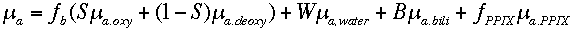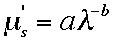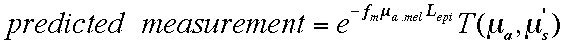# Spectral analysis

The analysis of spectra was accomplished by least squares fitting for the following parameters:

• fb: Volume fraction of blood
• S: Mixed arteriovenous oxygen saturation based on hemoglobin absorption
• W: Volume fraction of water
• B. Amount of bilirubin modeled as a Gaussian centered at 470 nm

PPIX: For the case of horse sarcoids that had received injections of the precursor ALA which was metabolically converted into protoporphyrin IX (PPIX), the absorption of PPIX contributed to the apparent bilirubin content and added to absorption at 630 nm.

W: Unless the spectrometer measured above 900 nm, it was difficult to specify a reliable water content (W). In our work, we simply assigned a typical value (W = 0.75) to the tissue rather than fitting for W.

The total absorption coefficient of the tissue wasa,b: The scattering properties were specified by fitting for factors a and b such thatfm: For the case of skin, the volume fraction of melanin in typical 60-um-thick epidermis (fm) was specified by fitting, such thatwhere Lepi equals twice the epidermal thickness (2 x 60 microns = 120 microns), fmel is the volume fraction of melanosomes in the epidermis, and ua.mel is the absorption coefficient of a melanosome = (6.6 x 10^11)(nm^(-3.33)) [cm^-1] where nm denotes wavelengths in nm (see skin optics). The factor of 2 is due to the double pass IN/OUT as photons enter then exit the skin.

In summary, spectra were analyzed so that measurement matched predicted measurement to yield the following values:

• fb
• S
• W
• B
• PPIX
• a, b
• fm